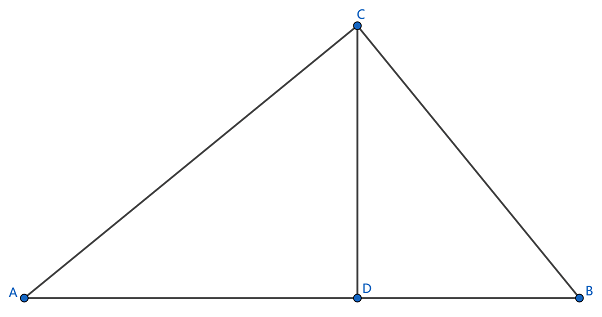安卓手机扫描二维码安装App

﻿$\triangle ABC$﻿﻿$\angle A$﻿﻿$\angle B$﻿均为锐角，﻿$AC=6$﻿﻿$BC=3\sqrt{3}$﻿ ，且﻿$\sin A =\dfrac{\sqrt{3}}{3}$﻿，则 ﻿$\cos B$﻿ 的值为(  ).

A. ﻿$\dfrac{5}{3}$﻿ B. ﻿$\dfrac{3}{5}$﻿

C. ﻿$\dfrac{\sqrt{5}}{3}$﻿ D. ﻿$\dfrac{\sqrt{3}}{5}$﻿A明显不对，因为根据定义cos A 等于邻边比斜边，而直角三角形中，斜边必大于邻边，所以\cos A必小于1. 正弦同理.苹果手机扫描二维码安装App